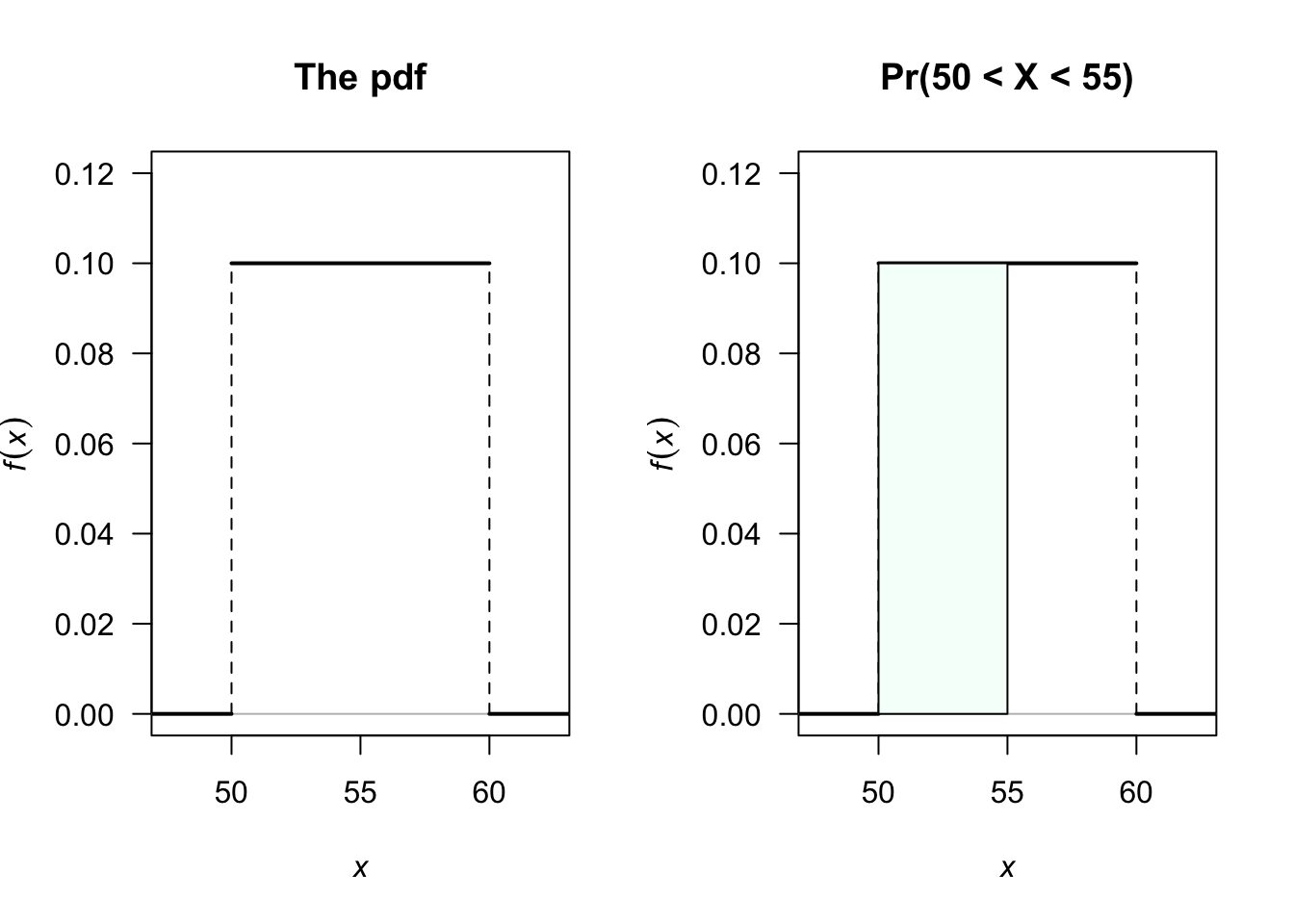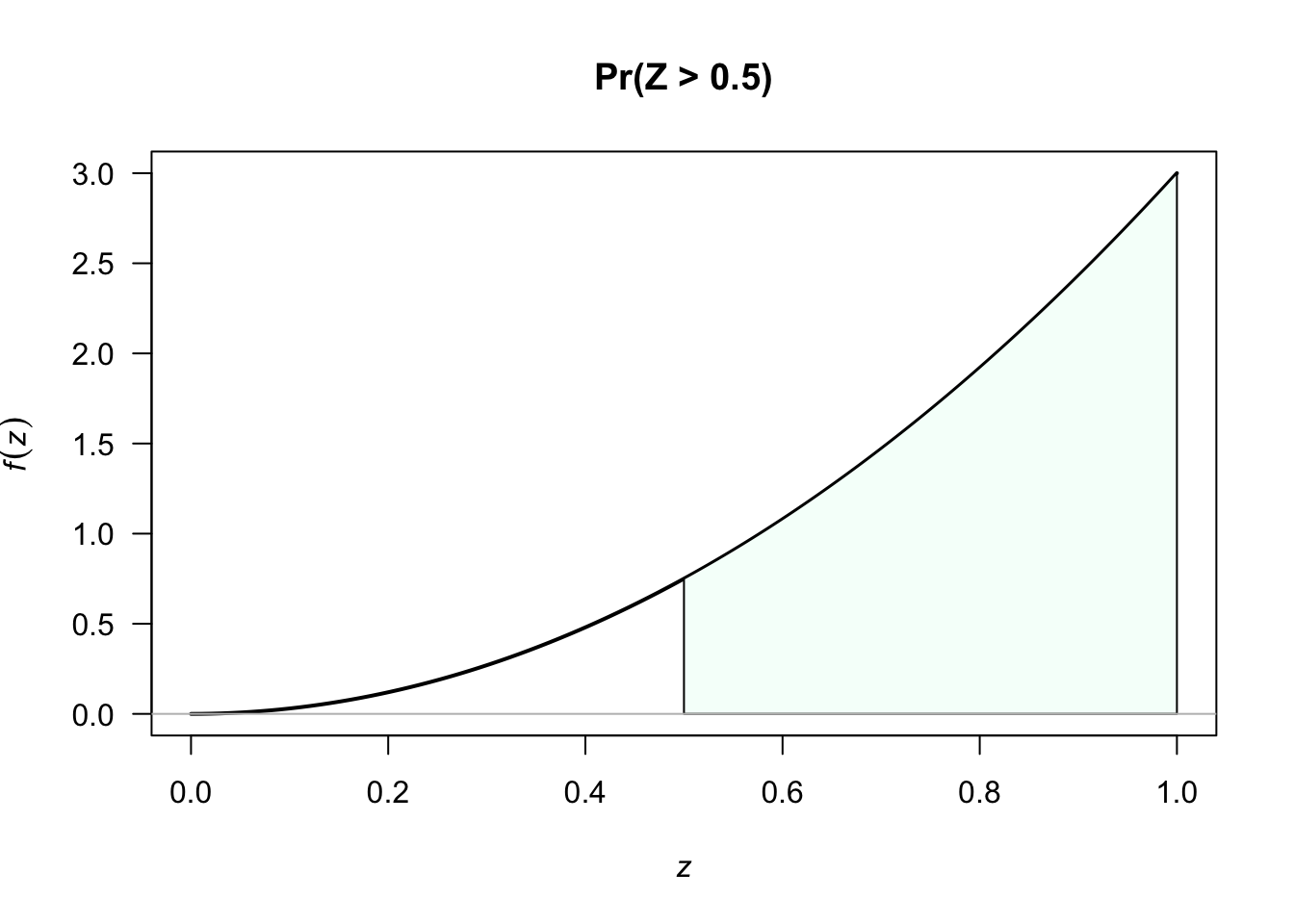## 2.1 Probability functions for continuous random variables

For continuous random variables, the probability function is called a probability density function (PDF).

Example 2.1 (Probability density function) A rv $$X$$ has the uniform continuous distribution defined from $$a$$ to $$b$$ if the PDF is $f_X(x) = \left\{ \begin{array}{ll} \displaystyle \frac{1}{b-a} & \text{if a<x<b};\\[6pt] 0 & \text{otherwise}. \end{array} \right.$ This is usually written, for convenience, as $f_X(x) = \displaystyle \frac{1}{b-a}\quad \text{for a<x<b}.$

A PDF, say $$f_X(x)$$ (or just $$f(x)$$ when there is no ambiguity), has two properties:

1. $$\displaystyle\int_{-\infty}^{\infty} f_X(x)\, dx = 1$$; that is, the total probability is one (in other words, $$x$$ certainly must take some value).
2. $$f_X(x) \ge 0$$ for all $$x$$; that is, probabilities are non-negative.

Probabilities for continuous rvs are found by integrating over an appropriate area.

Example 2.2 (Uniform continuous distribution) A rv $$X$$ has the uniform continuous distribution defined from $$50$$ to $$60$$ if the PDF is $f_X(x) = \displaystyle \frac{1}{60-50} = \frac{1}{10} \quad\text{for 50<x<60};$ see Fig. 2.1 (left panel). The probability that the  $$X$$ takes a value between $$50$$ and $$55$$ is $\Pr(50<X<55) = \int_{50}^{55} f_X(x)\, dx = \int_{50}^{55} \frac{1}{10}\, dx = 0.5;$
see Fig. 2.1 (right panel). Note that the probability of observing any value exactly is zero since $$X$$ is continuous.FIGURE 2.1: Left panel: A continuous uniform distribution defined for $$50<x<60$$. Right panel: Displaying the probability that the value of $$X$$ is between 50 and 55.

Example 2.3 (PDF: Continuous rv) Consider a rv $$Z$$ with PDF $f_Z(z) = 3 z^2\quad\text{for 0<z<1}.$ The probability that $$z>0.5$$ is $\Pr(Z>0.5) = 3 \int_{1/2}^{1} z^2 \, dz = z^3 \bigg\rvert_{z=1/2}^{z=1} = 1 - \frac{1}{8} = \frac{7}{8};$ see Fig.2.2.FIGURE 2.2: The PDF for the rv $$Z$$

Note that for $$X$$ continuous:

1. $$\Pr(X=x) = 0$$ for all values of $$x$$.
2. Hence, $$\Pr(a < X < b) = \Pr( a \le X < b) = \Pr(a < X \le b) = \Pr(a\le X\le b)$$.